Algebra and Trigonometry 2e

# Chapter 12

### 12.1The Ellipse

1.

$x 2 + y 2 16 =1 x 2 + y 2 16 =1$

2.

$( x−1 ) 2 16 + ( y−3 ) 2 4 =1 ( x−1 ) 2 16 + ( y−3 ) 2 4 =1$

3.

center: $( 0,0 ); ( 0,0 );$ vertices: $( ±6,0 ); ( ±6,0 );$ co-vertices: $( 0,±2 ); ( 0,±2 );$ foci: $( ±4 2 ,0 ) ( ±4 2 ,0 )$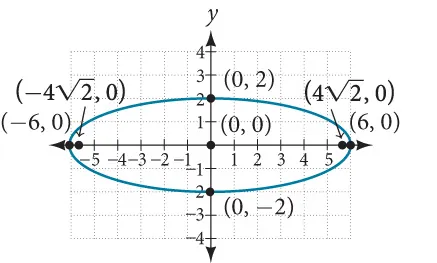4.

Standard form: $x 2 16 + y 2 49 =1; x 2 16 + y 2 49 =1;$ center: $( 0,0 ); ( 0,0 );$ vertices: $( 0,±7 ); ( 0,±7 );$ co-vertices: $( ±4,0 ); ( ±4,0 );$ foci: $( 0,± 33 ) ( 0,± 33 )$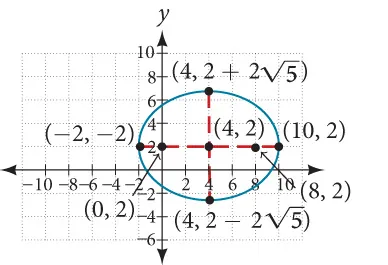5.

Center: $( 4,2 ); ( 4,2 );$ vertices: $( −2,2 ) ( −2,2 )$ and $( 10,2 ); ( 10,2 );$ co-vertices: $( 4,2−2 5 ) ( 4,2−2 5 )$ and $( 4,2+2 5 ); ( 4,2+2 5 );$ foci: $( 0,2 ) ( 0,2 )$ and $( 8,2 ) ( 8,2 )$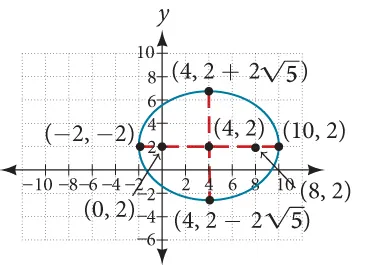6.

$(x−3) 2 4 + ( y+1 ) 2 16 =1; (x−3) 2 4 + ( y+1 ) 2 16 =1;$ center: $( 3,−1 ); ( 3,−1 );$ vertices: $( 3,−5 ) ( 3,−5 )$ and $( 3,3 ); ( 3,3 );$ co-vertices: $( 1,−1 ) ( 1,−1 )$ and $( 5,−1 ); ( 5,−1 );$ foci: $( 3,−1−2 3 ) ( 3,−1−2 3 )$ and $( 3,−1+2 3 ) ( 3,−1+2 3 )$

7.
1. $x 2 57,600 + y 2 25,600 =1 x 2 57,600 + y 2 25,600 =1$
2. The people are standing 358 feet apart.

### 12.2The Hyperbola

1.

Vertices: $( ±3,0 ); ( ±3,0 );$ Foci: $( ± 34 ,0 ) ( ± 34 ,0 )$

2.

$y 2 4 − x 2 16 =1 y 2 4 − x 2 16 =1$

3.

$( y−3 ) 2 25 - ( x−1 ) 2 144 =1 ( y−3 ) 2 25 - ( x−1 ) 2 144 =1$

4.

vertices: $( ±12,0 ); ( ±12,0 );$ co-vertices: $( 0,±9 ); ( 0,±9 );$ foci: $( ±15,0 ); ( ±15,0 );$ asymptotes: $y=± 3 4 x; y=± 3 4 x;$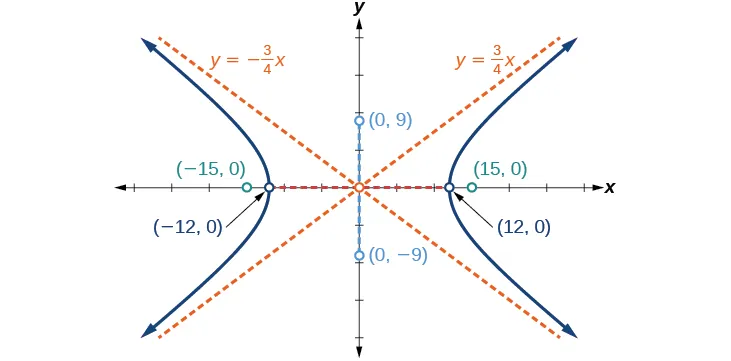5.

center: $( 3,−4 ); ( 3,−4 );$ vertices: $( 3,−14 ) ( 3,−14 )$ and $( 3,6 ); ( 3,6 );$ co-vertices: $( −5,−4 ); ( −5,−4 );$ and $( 11,−4 ); ( 11,−4 );$ foci: $( 3,−4−2 41 ) ( 3,−4−2 41 )$ and $( 3,−4+2 41 ); ( 3,−4+2 41 );$ asymptotes: $y=± 5 4 ( x−3 )−4 y=± 5 4 ( x−3 )−4$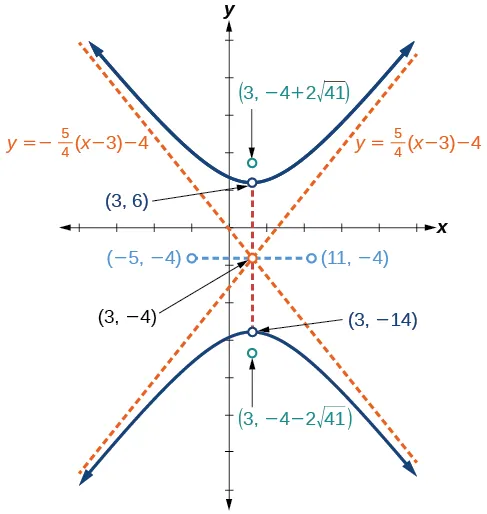6.

The sides of the tower can be modeled by the hyperbolic equation.

### 12.3The Parabola

1.

Focus: $( −4,0 ); ( −4,0 );$ Directrix: $x=4; x=4;$ Endpoints of the latus rectum: $( −4,±8 ) ( −4,±8 )$2.

Focus: $( 0,2 ); ( 0,2 );$ Directrix: $y=−2; y=−2;$ Endpoints of the latus rectum: $( ±4,2 ). ( ±4,2 ).$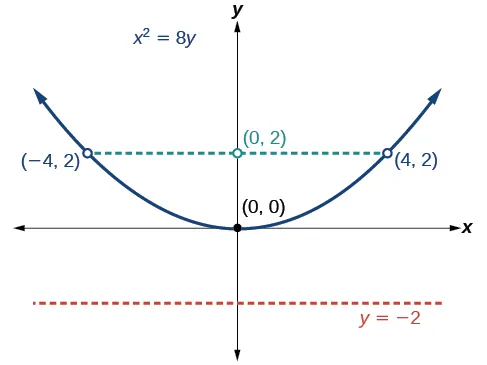3.

$x 2 =14y. x 2 =14y.$

4.

Vertex: $( 8,−1 ); ( 8,−1 );$ Axis of symmetry: $y=−1; y=−1;$ Focus: $( 9,−1 ); ( 9,−1 );$ Directrix: $x=7; x=7;$ Endpoints of the latus rectum: $( 9,−3 ) ( 9,−3 )$ and $( 9,1 ). ( 9,1 ).$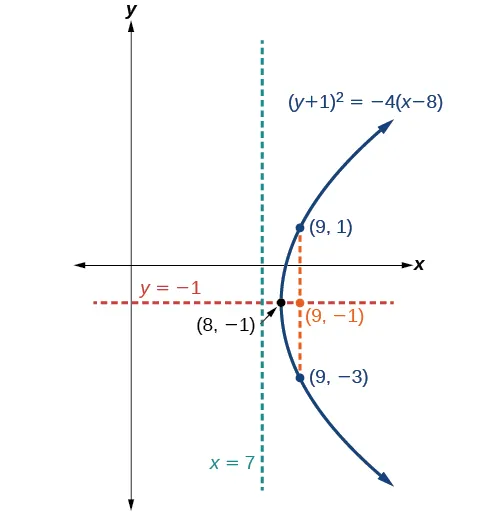5.

Vertex: $( −2,3 ); ( −2,3 );$ Axis of symmetry: $x=−2; x=−2;$ Focus: $( −2,−2 ); ( −2,−2 );$ Directrix: $y=8; y=8;$ Endpoints of the latus rectum: $( −12,−2 ) ( −12,−2 )$ and $( 8,−2 ). ( 8,−2 ).$6.
1. $y 2 =1280x y 2 =1280x$
2. The depth of the cooker is 500 mm

### 12.4Rotation of Axes

1.
1. hyperbola
2. ellipse
2.

$x ′ 2 4 + y ′ 2 1 =1 x ′ 2 4 + y ′ 2 1 =1$

3.
1. hyperbola
2. ellipse

### 12.5Conic Sections in Polar Coordinates

1.

ellipse; $e= 1 3 ;x=−2 e= 1 3 ;x=−2$

2.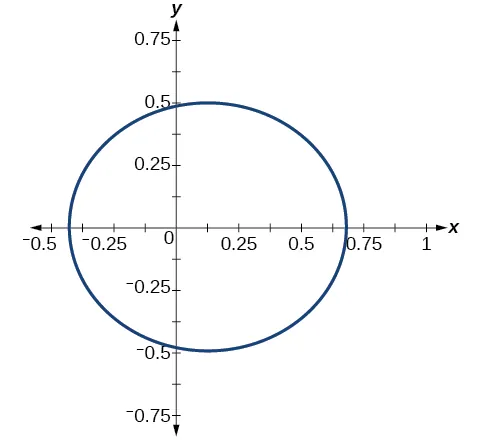3.

$r= 1 1−cosθ r= 1 1−cosθ$

4.

$4−8x+3 x 2 − y 2 =0 4−8x+3 x 2 − y 2 =0$

### 12.1 Section Exercises

1.

An ellipse is the set of all points in the plane the sum of whose distances from two fixed points, called the foci, is a constant.

3.

This special case would be a circle.

5.

It is symmetric about the x-axis, y-axis, and the origin.

7.

yes; $x 2 3 2 + y 2 2 2 =1 x 2 3 2 + y 2 2 2 =1$

9.

yes; $x 2 ( 1 2 ) 2 + y 2 ( 1 3 ) 2 =1 x 2 ( 1 2 ) 2 + y 2 ( 1 3 ) 2 =1$

11.

$x 2 2 2 + y 2 7 2 =1; x 2 2 2 + y 2 7 2 =1;$ Endpoints of major axis $( 0,7 ) ( 0,7 )$ and $( 0,−7 ). ( 0,−7 ).$ Endpoints of minor axis $( 2,0 ) ( 2,0 )$ and $( −2,0 ). ( −2,0 ).$ Foci at $( 0,3 5 ),( 0,−3 5 ). ( 0,3 5 ),( 0,−3 5 ).$

13.

$x 2 ( 1 ) 2 + y 2 ( 1 3 ) 2 =1; x 2 ( 1 ) 2 + y 2 ( 1 3 ) 2 =1;$ Endpoints of major axis $( 1,0 ) ( 1,0 )$ and $( −1,0 ). ( −1,0 ).$ Endpoints of minor axis $( 0, 1 3 ),( 0,− 1 3 ). ( 0, 1 3 ),( 0,− 1 3 ).$ Foci at $( 2 2 3 ,0 ),( − 2 2 3 ,0 ). ( 2 2 3 ,0 ),( − 2 2 3 ,0 ).$

15.

$( x−2 ) 2 7 2 + ( y−4 ) 2 5 2 =1; ( x−2 ) 2 7 2 + ( y−4 ) 2 5 2 =1;$ Endpoints of major axis $( 9,4 ),( −5,4 ). ( 9,4 ),( −5,4 ).$ Endpoints of minor axis $( 2,9 ),( 2,−1 ). ( 2,9 ),( 2,−1 ).$ Foci at $( 2+2 6 ,4 ),( 2−2 6 ,4 ). ( 2+2 6 ,4 ),( 2−2 6 ,4 ).$

17.

$( x+5 ) 2 2 2 + ( y−7 ) 2 3 2 =1; ( x+5 ) 2 2 2 + ( y−7 ) 2 3 2 =1;$ Endpoints of major axis $( −5,10 ),( −5,4 ). ( −5,10 ),( −5,4 ).$ Endpoints of minor axis $( −3,7 ),( −7,7 ). ( −3,7 ),( −7,7 ).$ Foci at $( −5,7+ 5 ),( −5,7− 5 ). ( −5,7+ 5 ),( −5,7− 5 ).$

19.

$( x−1 ) 2 3 2 + ( y−4 ) 2 2 2 =1; ( x−1 ) 2 3 2 + ( y−4 ) 2 2 2 =1;$ Endpoints of major axis $( 4,4 ),( −2,4 ). ( 4,4 ),( −2,4 ).$ Endpoints of minor axis $( 1,6 ),( 1,2 ). ( 1,6 ),( 1,2 ).$ Foci at $( 1+ 5 ,4 ),( 1− 5 ,4 ). ( 1+ 5 ,4 ),( 1− 5 ,4 ).$

21.

$( x−3 ) 2 ( 3 2 ) 2 + ( y−5 ) 2 ( 2 ) 2 =1; ( x−3 ) 2 ( 3 2 ) 2 + ( y−5 ) 2 ( 2 ) 2 =1;$ Endpoints of major axis $( 3+3 2 ,5 ),( 3−3 2 ,5 ). ( 3+3 2 ,5 ),( 3−3 2 ,5 ).$ Endpoints of minor axis $( 3,5+ 2 ),( 3,5− 2 ). ( 3,5+ 2 ),( 3,5− 2 ).$ Foci at $( 7,5 ),( −1,5 ). ( 7,5 ),( −1,5 ).$

23.

$( x+5 ) 2 ( 5 ) 2 + ( y−2 ) 2 ( 2 ) 2 =1; ( x+5 ) 2 ( 5 ) 2 + ( y−2 ) 2 ( 2 ) 2 =1;$ Endpoints of major axis $( 0,2 ),( −10,2 ). ( 0,2 ),( −10,2 ).$ Endpoints of minor axis $( −5,4 ),( −5,0 ). ( −5,4 ),( −5,0 ).$ Foci at $( −5+ 21 ,2 ),( −5− 21 ,2 ). ( −5+ 21 ,2 ),( −5− 21 ,2 ).$

25.

$( x+3 ) 2 ( 5 ) 2 + ( y+4 ) 2 ( 2 ) 2 =1; ( x+3 ) 2 ( 5 ) 2 + ( y+4 ) 2 ( 2 ) 2 =1;$ Endpoints of major axis $( 2,−4 ),( −8,−4 ). ( 2,−4 ),( −8,−4 ).$ Endpoints of minor axis $( −3,−2 ),( −3,−6 ). ( −3,−2 ),( −3,−6 ).$ Foci at $( −3+ 21 ,−4 ),( −3− 21 ,−4 ). ( −3+ 21 ,−4 ),( −3− 21 ,−4 ).$

27.

Foci $( −3,−1+ 11 ),( −3,−1− 11 ) ( −3,−1+ 11 ),( −3,−1− 11 )$

29.

Focus $( 0,0 ) ( 0,0 )$

31.

Foci $( −10,30 ),( −10,−30 ) ( −10,30 ),( −10,−30 )$

33.

Center $( 0,0 ), ( 0,0 ),$ Vertices $( 4,0 ),( −4,0 ),(0,3),(0,−3), ( 4,0 ),( −4,0 ),(0,3),(0,−3),$ Foci $( 7 ,0 ),( − 7 ,0 ) ( 7 ,0 ),( − 7 ,0 )$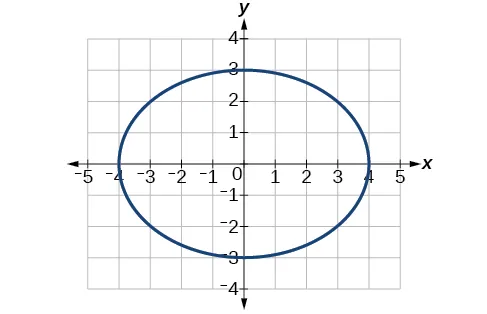35.

Center $( 0,0 ), ( 0,0 ),$ Vertices $( 1 9 ,0 ),( − 1 9 ,0 ),( 0, 1 7 ),( 0,− 1 7 ), ( 1 9 ,0 ),( − 1 9 ,0 ),( 0, 1 7 ),( 0,− 1 7 ),$ Foci $( 0, 4 2 63 ),( 0,− 4 2 63 ) ( 0, 4 2 63 ),( 0,− 4 2 63 )$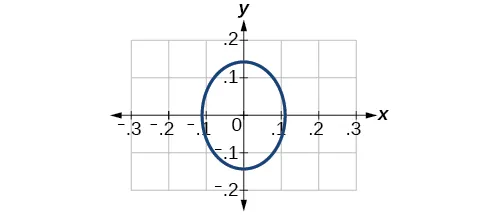37.

Center $( −3,3 ), ( −3,3 ),$ Vertices $( 0,3 ),( −6,3 ),( −3,0 ),( −3,6 ), ( 0,3 ),( −6,3 ),( −3,0 ),( −3,6 ),$ Focus $( −3,3 ) ( −3,3 )$

Note that this ellipse is a circle. The circle has only one focus, which coincides with the center.39.

Center $( 1,1 ), ( 1,1 ),$ Vertices $( 5,1 ),( −3,1 ),( 1,3 ),( 1,−1 ), ( 5,1 ),( −3,1 ),( 1,3 ),( 1,−1 ),$ Foci $( 1,1+2 3 ),( 1,1−2 3 ) ( 1,1+2 3 ),( 1,1−2 3 )$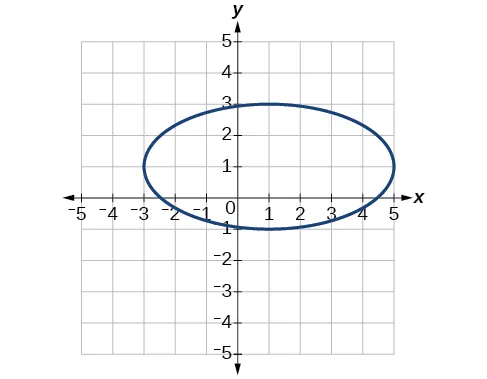41.

Center $( −4,5 ), ( −4,5 ),$ Vertices $( −2,5 ),( −6,4 ),( −4,6 ),( −4,4 ), ( −2,5 ),( −6,4 ),( −4,6 ),( −4,4 ),$ Foci $( −4+ 3 ,5 ),( −4− 3 ,5 ) ( −4+ 3 ,5 ),( −4− 3 ,5 )$43.

Center $( −2,1 ), ( −2,1 ),$ Vertices $( 0,1 ),( −4,1 ),( −2,5 ),( −2,−3 ), ( 0,1 ),( −4,1 ),( −2,5 ),( −2,−3 ),$ Foci $( −2,1+2 3 ),( −2,1−2 3 ) ( −2,1+2 3 ),( −2,1−2 3 )$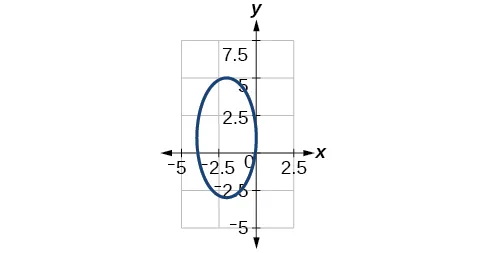45.

Center $( −2,−2 ), ( −2,−2 ),$ Vertices $( 0,−2 ),( −4,−2 ),( −2,0 ),( −2,−4 ), ( 0,−2 ),( −4,−2 ),( −2,0 ),( −2,−4 ),$ Focus $( −2,−2 ) ( −2,−2 )$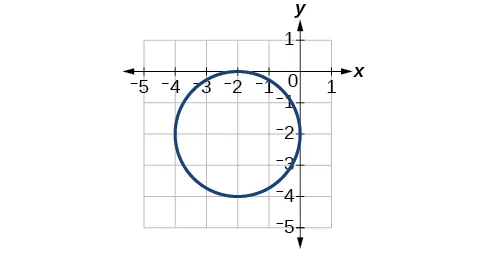47.

$x 2 25 + y 2 29 =1 x 2 25 + y 2 29 =1$

49.

$( x−4 ) 2 25 + ( y−2 ) 2 1 =1 ( x−4 ) 2 25 + ( y−2 ) 2 1 =1$

51.

$( x+3 ) 2 16 + ( y−4 ) 2 4 =1 ( x+3 ) 2 16 + ( y−4 ) 2 4 =1$

53.

$x 2 81 + y 2 9 =1 x 2 81 + y 2 9 =1$

55.

$( x+2 ) 2 4 + ( y−2 ) 2 9 =1 ( x+2 ) 2 4 + ( y−2 ) 2 9 =1$

57.

59.

square units.

61.

square units.

63.

$x 2 4 h 2 + y 2 1 4 h 2 =1 x 2 4 h 2 + y 2 1 4 h 2 =1$

65.

$x 2 400 + y 2 144 =1 x 2 400 + y 2 144 =1$ . Distance = 17.32 feet

67.

Approximately 51.96 feet

### 12.2 Section Exercises

1.

A hyperbola is the set of points in a plane the difference of whose distances from two fixed points (foci) is a positive constant.

3.

The foci must lie on the transverse axis and be in the interior of the hyperbola.

5.

The center must be the midpoint of the line segment joining the foci.

7.

yes $x 2 6 2 − y 2 3 2 =1 x 2 6 2 − y 2 3 2 =1$

9.

yes $x 2 4 2 − y 2 5 2 =1 x 2 4 2 − y 2 5 2 =1$

11.

$x 2 5 2 − y 2 6 2 =1; x 2 5 2 − y 2 6 2 =1;$ vertices: $( 5,0 ),( −5,0 ); ( 5,0 ),( −5,0 );$ foci: $( 61 ,0 ),( − 61 ,0 ); ( 61 ,0 ),( − 61 ,0 );$ asymptotes: $y= 6 5 x,y=− 6 5 x y= 6 5 x,y=− 6 5 x$

13.

$y 2 2 2 − x 2 9 2 =1; y 2 2 2 − x 2 9 2 =1;$ vertices: $( 0,2 ),( 0,−2 ); ( 0,2 ),( 0,−2 );$ foci: $( 0, 85 ),( 0,− 85 ); ( 0, 85 ),( 0,− 85 );$ asymptotes: $y= 2 9 x,y=− 2 9 x y= 2 9 x,y=− 2 9 x$

15.

$( x−1 ) 2 3 2 − ( y−2 ) 2 4 2 =1; ( x−1 ) 2 3 2 − ( y−2 ) 2 4 2 =1;$ vertices: $( 4,2 ),( −2,2 ); ( 4,2 ),( −2,2 );$ foci: $( 6,2 ),( −4,2 ); ( 6,2 ),( −4,2 );$ asymptotes: $y= 4 3 ( x−1 )+2,y=− 4 3 ( x−1 )+2 y= 4 3 ( x−1 )+2,y=− 4 3 ( x−1 )+2$

17.

$( x−2 ) 2 7 2 − ( y+7 ) 2 7 2 =1; ( x−2 ) 2 7 2 − ( y+7 ) 2 7 2 =1;$ vertices: $( 9,−7 ),( −5,−7 ); ( 9,−7 ),( −5,−7 );$ foci: $( 2+7 2 ,−7 ),( 2−7 2 ,−7 ); ( 2+7 2 ,−7 ),( 2−7 2 ,−7 );$ asymptotes: $y=x−9,y=−x−5 y=x−9,y=−x−5$

19.

$( x+3 ) 2 3 2 − ( y−3 ) 2 3 2 =1; ( x+3 ) 2 3 2 − ( y−3 ) 2 3 2 =1;$ vertices: $( 0,3 ),( −6,3 ); ( 0,3 ),( −6,3 );$ foci: $( −3+3 2 ,1 ),( −3−3 2 ,1 ); ( −3+3 2 ,1 ),( −3−3 2 ,1 );$ asymptotes: $y=x+6,y=−x y=x+6,y=−x$

21.

$( y−4 ) 2 2 2 − ( x−3 ) 2 4 2 =1; ( y−4 ) 2 2 2 − ( x−3 ) 2 4 2 =1;$ vertices: $( 3,6 ),( 3,2 ); ( 3,6 ),( 3,2 );$ foci: $( 3,4+2 5 ),( 3,4−2 5 ); ( 3,4+2 5 ),( 3,4−2 5 );$ asymptotes: $y= 1 2 ( x−3 )+4,y=− 1 2 ( x−3 )+4 y= 1 2 ( x−3 )+4,y=− 1 2 ( x−3 )+4$

23.

$( y+5 ) 2 7 2 − ( x+1 ) 2 70 2 =1; ( y+5 ) 2 7 2 − ( x+1 ) 2 70 2 =1;$ vertices: $( −1,2 ),( −1,−12 ); ( −1,2 ),( −1,−12 );$ foci: $( −1,−5+7 101 ),( −1,−5−7 101 ); ( −1,−5+7 101 ),( −1,−5−7 101 );$ asymptotes: $y= 1 10 ( x+1 )−5,y=− 1 10 ( x+1 )−5 y= 1 10 ( x+1 )−5,y=− 1 10 ( x+1 )−5$

25.

$( x+3 ) 2 5 2 − ( y−4 ) 2 2 2 =1; ( x+3 ) 2 5 2 − ( y−4 ) 2 2 2 =1;$ vertices: $( 2,4 ),( −8,4 ); ( 2,4 ),( −8,4 );$ foci: $( −3+ 29 ,4 ),( −3− 29 ,4 ); ( −3+ 29 ,4 ),( −3− 29 ,4 );$ asymptotes: $y= 2 5 ( x+3 )+4,y=− 2 5 ( x+3 )+4 y= 2 5 ( x+3 )+4,y=− 2 5 ( x+3 )+4$

27.

$y= 2 5 ( x−3 )−4,y=− 2 5 ( x−3 )−4 y= 2 5 ( x−3 )−4,y=− 2 5 ( x−3 )−4$

29.

$y= 3 4 ( x−1 )+1,y=− 3 4 ( x−1 )+1 y= 3 4 ( x−1 )+1,y=− 3 4 ( x−1 )+1$

31.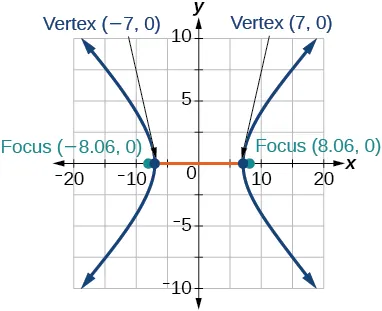33.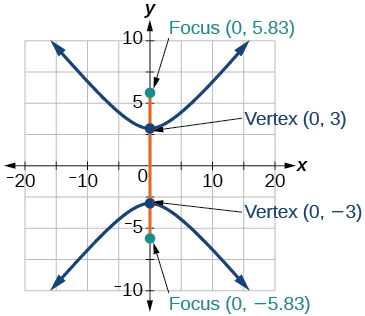35.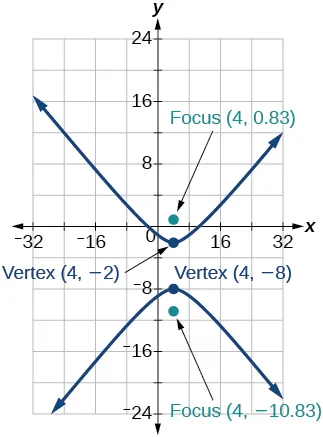37.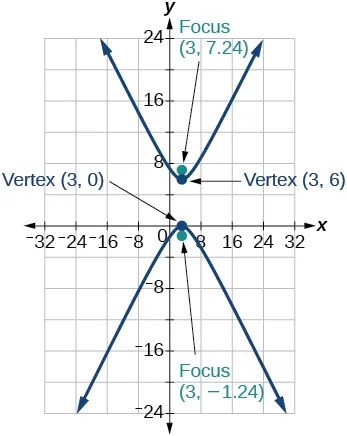39.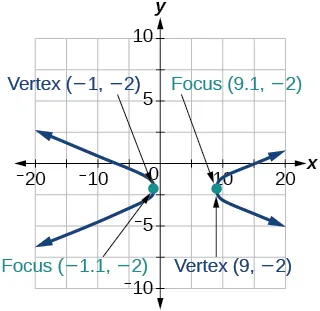41.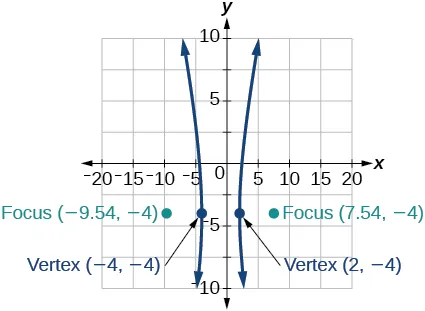43.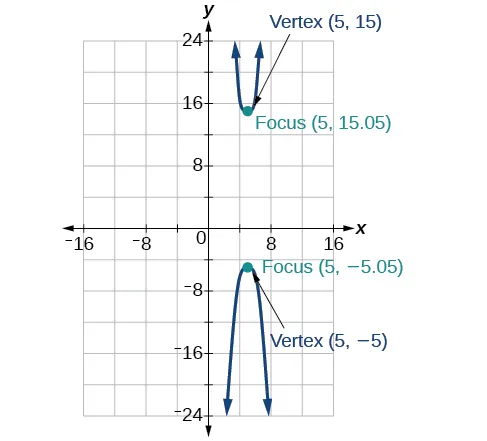45.

$x 2 9 − y 2 16 =1 x 2 9 − y 2 16 =1$

47.

$( x−6 ) 2 25 − ( y−1 ) 2 11 =1 ( x−6 ) 2 25 − ( y−1 ) 2 11 =1$

49.

$( x−4 ) 2 25 − ( y−2 ) 2 1 =1 ( x−4 ) 2 25 − ( y−2 ) 2 1 =1$

51.

$y 2 16 − x 2 25 =1 y 2 16 − x 2 25 =1$

53.

$y 2 9 − ( x+1 ) 2 9 =1 y 2 9 − ( x+1 ) 2 9 =1$

55.

$( x+3 ) 2 25 − ( y+3 ) 2 25 =1 ( x+3 ) 2 25 − ( y+3 ) 2 25 =1$

57.

$y( x )=3 x 2 +1 ,y( x )=−3 x 2 +1 y( x )=3 x 2 +1 ,y( x )=−3 x 2 +1$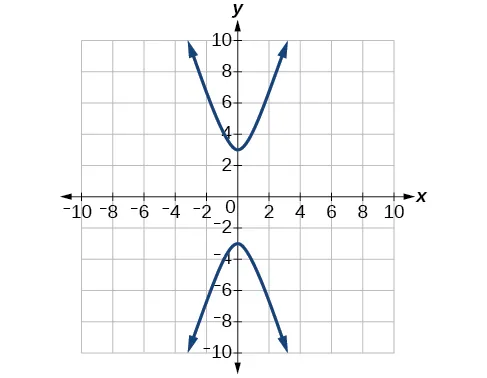59.

$y( x )=1+2 x 2 +4x+5 ,y( x )=1−2 x 2 +4x+5 y( x )=1+2 x 2 +4x+5 ,y( x )=1−2 x 2 +4x+5$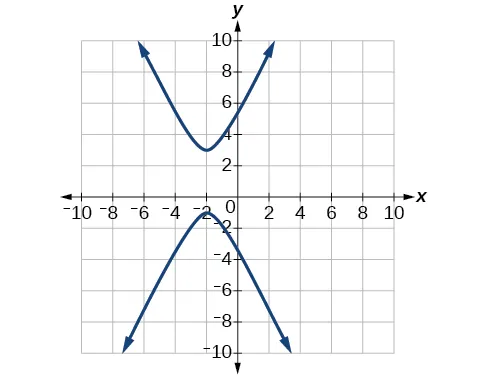61.

$x 2 25 − y 2 25 =1 x 2 25 − y 2 25 =1$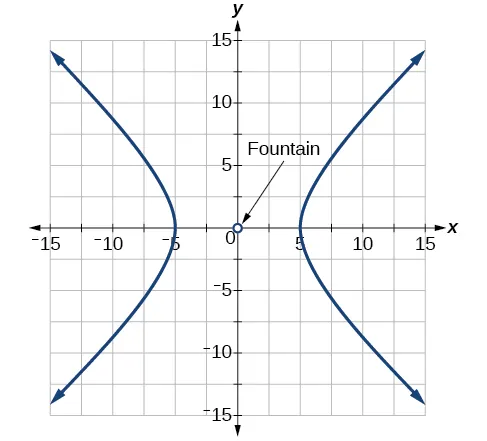63.

$x 2 100 − y 2 25 =1 x 2 100 − y 2 25 =1$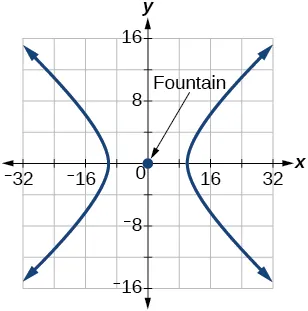65.

$x 2 400 − y 2 225 =1 x 2 400 − y 2 225 =1$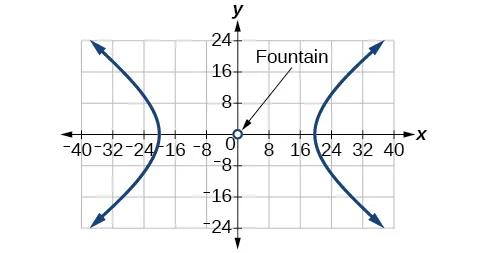67.

$4(x-1)2-y22=16 4(x-1)2-y22=16$

69.

$( x−h ) 2 a2 - (y-k)2 b2 =(x-3)2-9y2=4 ( x−h ) 2 a2 -(y-k)2 b2=(x-3)2-9y2=4$

### 12.3 Section Exercises

1.

A parabola is the set of points in the plane that lie equidistant from a fixed point, the focus, and a fixed line, the directrix.

3.

The graph will open down.

5.

The distance between the focus and directrix will increase.

7.

yes $x2=4(116)y x2=4(116)y$

9.

yes $( y−3 ) 2 =4(2)( x−2 ) ( y−3 ) 2 =4(2)( x−2 )$

11.

$y 2 = 1 8 x,V:(0,0);F:( 1 32 ,0 );d:x=− 1 32 y 2 = 1 8 x,V:(0,0);F:( 1 32 ,0 );d:x=− 1 32$

13.

$x 2 =− 1 4 y,V:( 0,0 );F:( 0,− 1 16 );d:y= 1 16 x 2 =− 1 4 y,V:( 0,0 );F:( 0,− 1 16 );d:y= 1 16$

15.

$y 2 = 1 36 x,V:( 0,0 );F:( 1 144 ,0 );d:x=− 1 144 y 2 = 1 36 x,V:( 0,0 );F:( 1 144 ,0 );d:x=− 1 144$

17.

$( x−1 ) 2 =4( y−1 ),V:( 1,1 );F:( 1,2 );d:y=0 ( x−1 ) 2 =4( y−1 ),V:( 1,1 );F:( 1,2 );d:y=0$

19.

$( y−4 ) 2 =2( x+3 ),V:( −3,4 );F:( − 5 2 ,4 );d:x=− 7 2 ( y−4 ) 2 =2( x+3 ),V:( −3,4 );F:( − 5 2 ,4 );d:x=− 7 2$

21.

$( x+4 ) 2 =24( y+1 ),V:( −4,−1 );F:( −4,5 );d:y=−7 ( x+4 ) 2 =24( y+1 ),V:( −4,−1 );F:( −4,5 );d:y=−7$

23.

$( y−3 ) 2 =−12( x+1 ),V:( −1,3 );F:( −4,3 );d:x=2 ( y−3 ) 2 =−12( x+1 ),V:( −1,3 );F:( −4,3 );d:x=2$

25.

$( x−5 ) 2 = 4 5 ( y+3 ),V:( 5,−3 );F:( 5,− 14 5 );d:y=− 16 5 ( x−5 ) 2 = 4 5 ( y+3 ),V:( 5,−3 );F:( 5,− 14 5 );d:y=− 16 5$

27.

$( x−2 ) 2 =−2( y−5 ),V:( 2,5 );F:( 2, 9 2 );d:y= 11 2 ( x−2 ) 2 =−2( y−5 ),V:( 2,5 );F:( 2, 9 2 );d:y= 11 2$

29.

$( y−1 ) 2 = 4 3 ( x−5 ),V:( 5,1 );F:( 16 3 ,1 );d:x= 14 3 ( y−1 ) 2 = 4 3 ( x−5 ),V:( 5,1 );F:( 16 3 ,1 );d:x= 14 3$

31.33.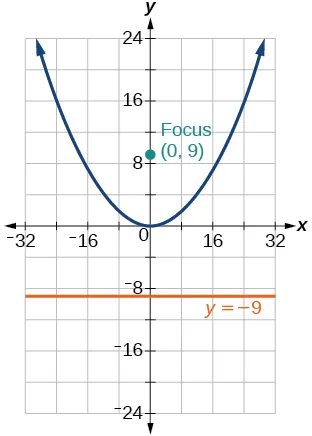35.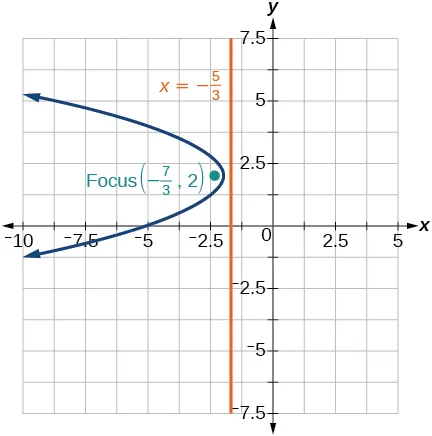37.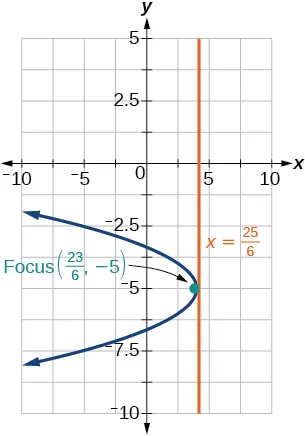39.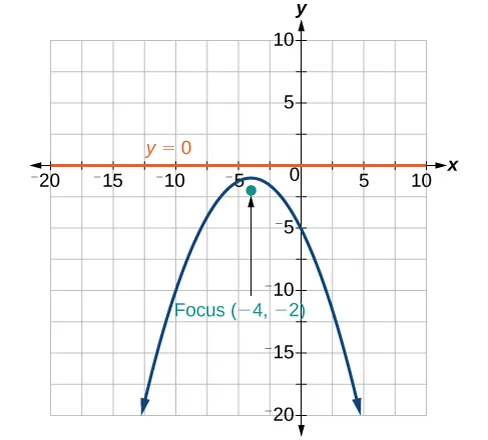41.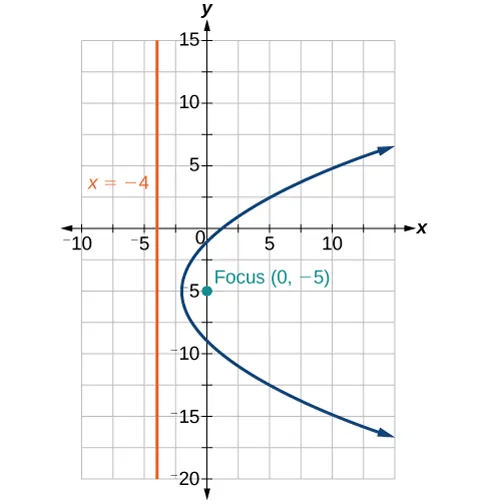43.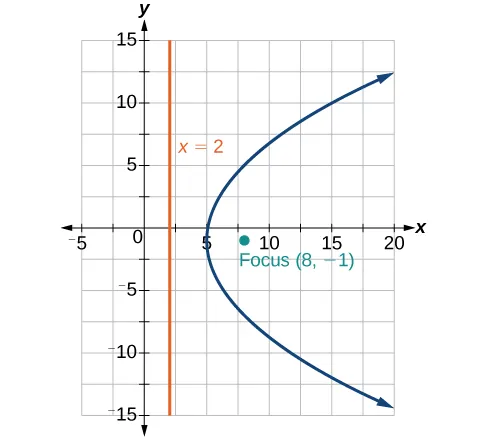45.

$x 2 =−16y x 2 =−16y$

47.

$( y−2 ) 2 =4 2 ( x−2 ) ( y−2 ) 2 =4 2 ( x−2 )$

49.

$( y+ 3 ) 2 =−4 2 ( x− 2 ) ( y+ 3 ) 2 =−4 2 ( x− 2 )$

51.

$x 2 =y x 2 =y$

53.

$( y−2 ) 2 = 1 4 ( x+2 ) ( y−2 ) 2 = 1 4 ( x+2 )$

55.

$( y− 3 ) 2 =4 5 ( x+ 2 ) ( y− 3 ) 2 =4 5 ( x+ 2 )$

57.

$y 2 =−8x y 2 =−8x$

59.

$( y+1 ) 2 =12( x+3 ) ( y+1 ) 2 =12( x+3 )$

61.

$( 0,1 ) ( 0,1 )$

63.

At the point 2.25 feet above the vertex.

65.

0.5625 feet

67.

$x 2 =−125( y−20 ), x 2 =−125( y−20 ),$ height is 7.2 feet

69.

2304 feet

### 12.4 Section Exercises

1.

The $xy xy$ term causes a rotation of the graph to occur.

3.

The conic section is a hyperbola.

5.

It gives the angle of rotation of the axes in order to eliminate the $xy xy$ term.

7.

$AB=0, AB=0,$ parabola

9.

$AB=−4<0, AB=−4<0,$ hyperbola

11.

$AB=6>0, AB=6>0,$ ellipse

13.

$B 2 −4AC=0, B 2 −4AC=0,$ parabola

15.

$B 2 −4AC=0, B 2 −4AC=0,$ parabola

17.

$B 2 −4AC=−96<0, B 2 −4AC=−96<0,$ ellipse

19.

$7 x ′ 2 +9 y ′ 2 −4=0 7 x ′ 2 +9 y ′ 2 −4=0$

21.

$3 x ′ 2 +2 x ′ y ′ −5 y ′ 2 +1=0 3 x ′ 2 +2 x ′ y ′ −5 y ′ 2 +1=0$

23.

$θ= 60 ∘ ,11 x ′ 2 − y ′ 2 + 3 x ′ + y ′ −4=0 θ= 60 ∘ ,11 x ′ 2 − y ′ 2 + 3 x ′ + y ′ −4=0$

25.

$θ= - 30 ∘ ,21 x ′ 2 +9 y ′ 2 +4 x ′ −4 3 y ′ −6=0 θ= - 30 ∘ ,21 x ′ 2 +9 y ′ 2 +4 x ′ −4 3 y ′ −6=0$

27.

$θ≈ 36.9 ∘ ,125 x ′ 2 +6 x ′ −42 y ′ +10=0 θ≈ 36.9 ∘ ,125 x ′ 2 +6 x ′ −42 y ′ +10=0$

29.

$θ= 45 ∘ ,3 x ′ 2 − y ′ 2 − 2 x ′ + 2 y ′ +1=0 θ= 45 ∘ ,3 x ′ 2 − y ′ 2 − 2 x ′ + 2 y ′ +1=0$

31.

$2 2 ( x ′ + y ′ )= 1 2 ( x ′ − y ′ ) 2 2 2 ( x ′ + y ′ )= 1 2 ( x ′ − y ′ ) 2$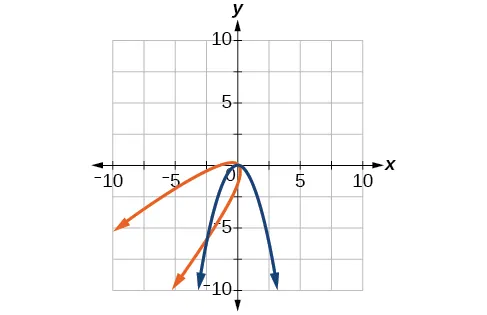33.

$( x ′ − y ′ ) 2 8 + ( x ′ + y ′ ) 2 2 =1 ( x ′ − y ′ ) 2 8 + ( x ′ + y ′ ) 2 2 =1$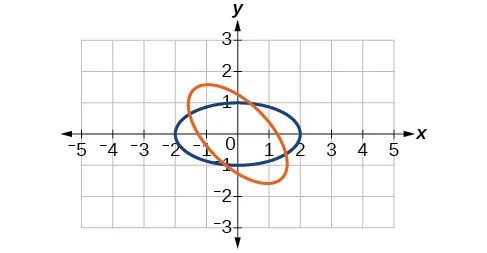35.

$( x ′ + y ′ ) 2 2 − ( x ′ − y ′ ) 2 2 =1 ( x ′ + y ′ ) 2 2 − ( x ′ − y ′ ) 2 2 =1$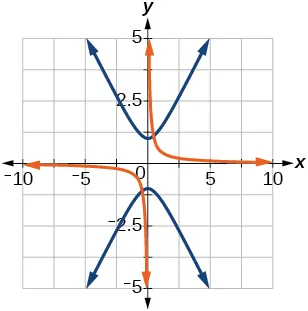37.

$3 2 x ′ − 1 2 y ′ = ( 1 2 x ′ + 3 2 y ′ −1 ) 2 3 2 x ′ − 1 2 y ′ = ( 1 2 x ′ + 3 2 y ′ −1 ) 2$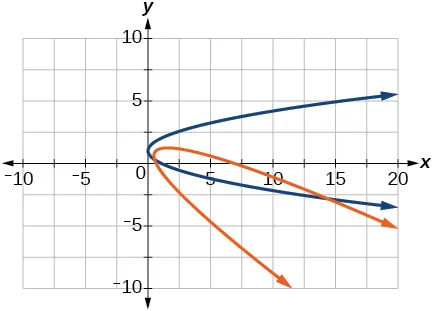39.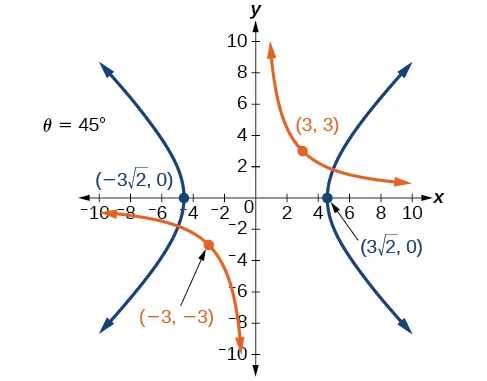41.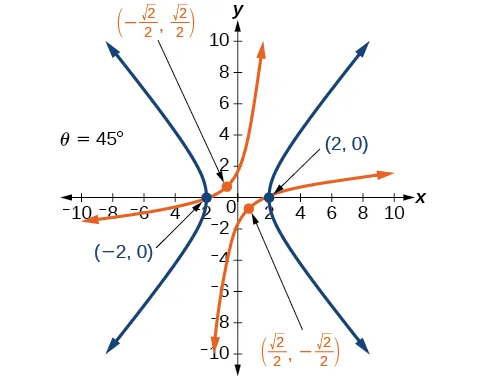43.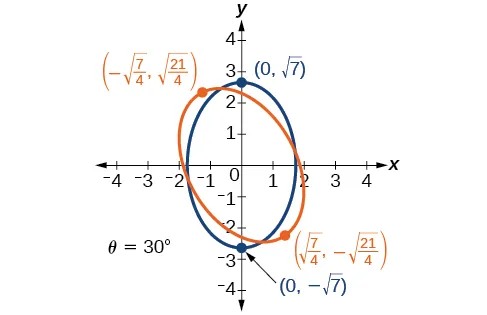45.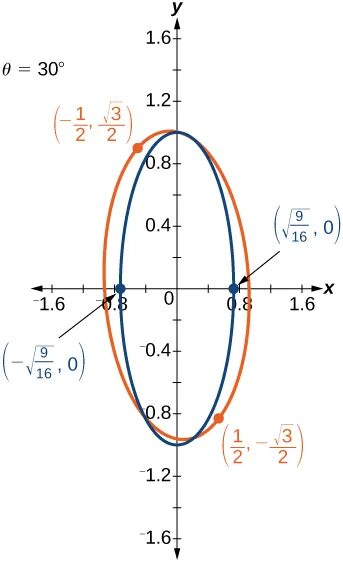47.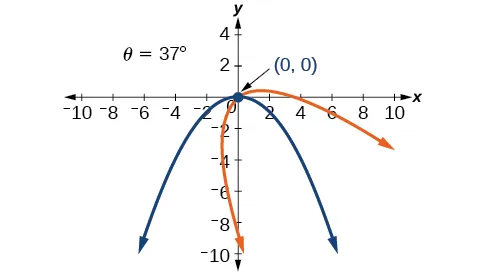49.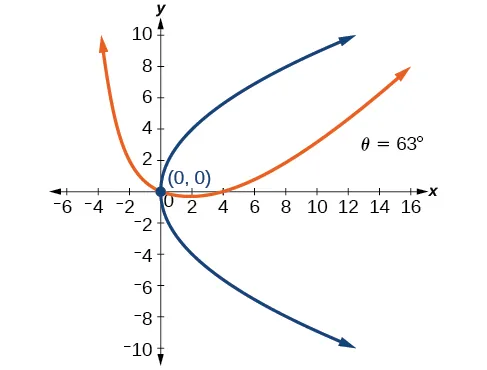51.

$θ= 45 ∘ θ= 45 ∘$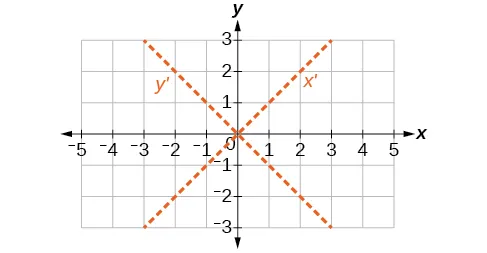53.

$θ= 60 ∘ θ= 60 ∘$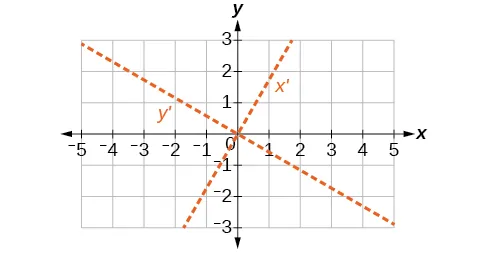55.

$θ≈ 36.9 ∘ θ≈ 36.9 ∘$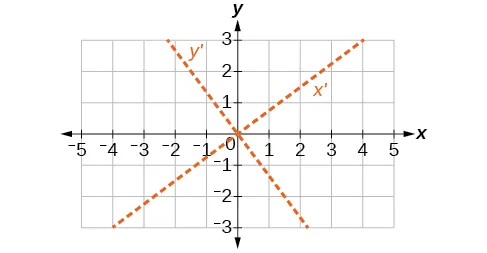57.

$−4 6

59.

$k=2 k=2$

### 12.5 Section Exercises

1.

If eccentricity is less than 1, it is an ellipse. If eccentricity is equal to 1, it is a parabola. If eccentricity is greater than 1, it is a hyperbola.

3.

The directrix will be parallel to the polar axis.

5.

One of the foci will be located at the origin.

7.

Parabola with $e=1 e=1$ and directrix $3 4 3 4$ units below the pole.

9.

Hyperbola with $e=2 e=2$ and directrix $5 2 5 2$ units above the pole.

11.

Parabola with $e=1 e=1$ and directrix $3 10 3 10$ units to the right of the pole.

13.

Ellipse with $e= 2 7 e= 2 7$ and directrix $2 2$ units to the right of the pole.

15.

Hyperbola with $e= 5 3 e= 5 3$ and directrix $11 5 11 5$ units above the pole.

17.

Hyperbola with $e= 8 7 e= 8 7$ and directrix $7 8 7 8$ units to the right of the pole.

19.

$25 x 2 +16 y 2 −12y−4=0 25 x 2 +16 y 2 −12y−4=0$

21.

$21 x 2 −4 y 2 −30x+9=0 21 x 2 −4 y 2 −30x+9=0$

23.

$64 y 2 =48x+9 64 y 2 =48x+9$

25.

$96 y 2 −25 x 2 +110y+25=0 96 y 2 −25 x 2 +110y+25=0$

27.

$3 x 2 +4 y 2 −2x−1=0 3 x 2 +4 y 2 −2x−1=0$

29.

$5 x 2 +9 y 2 −24x−36=0 5 x 2 +9 y 2 −24x−36=0$

31.33.35.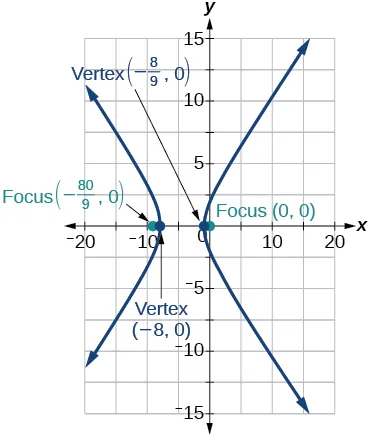37.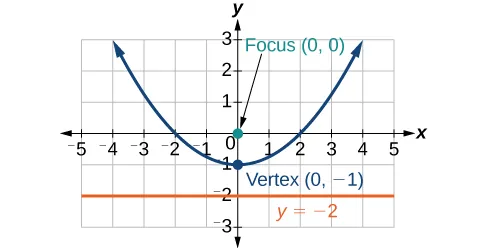39.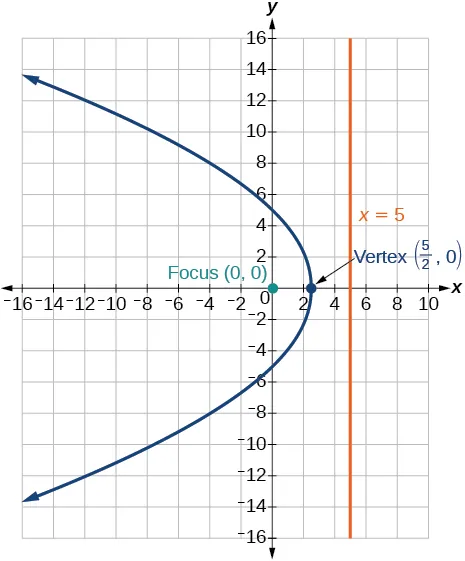41.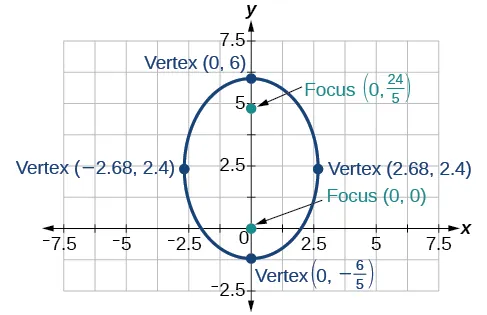43.

$r= 4 5+cosθ r= 4 5+cosθ$

45.

$r= 4 1+2sinθ r= 4 1+2sinθ$

47.

$r= 1 1+cosθ r= 1 1+cosθ$

49.

$r= 7 8−28cosθ r= 7 8−28cosθ$

51.

$r= 12 2+3sinθ r= 12 2+3sinθ$

53.

$r= 15 4−3cosθ r= 15 4−3cosθ$

55.

$r= 3 3−3cosθ r= 3 3−3cosθ$

57.

$r=± 2 1+sinθcosθ r=± 2 1+sinθcosθ$

59.

$r=± 2 4cosθ+3sinθ r=± 2 4cosθ+3sinθ$

### Review Exercises

1.

$x 2 5 2 + y 2 8 2 =1; x 2 5 2 + y 2 8 2 =1;$ center: $( 0,0 ); ( 0,0 );$ vertices: $( 5,0 ),( −5,0 ),( 0,8 ),( 0,−8 ); ( 5,0 ),( −5,0 ),( 0,8 ),( 0,−8 );$ foci: $( 0, 39 ),( 0,− 39 ) ( 0, 39 ),( 0,− 39 )$

3.

$(x+3) 2 1 2 + (y−2) 2 3 2 =1(−3,2);(−2,2),(−4,2),(−3,5),(−3,−1);( −3,2+2 2 ),( −3,2−2 2 ) (x+3) 2 1 2 + (y−2) 2 3 2 =1(−3,2);(−2,2),(−4,2),(−3,5),(−3,−1);( −3,2+2 2 ),( −3,2−2 2 )$

5.

center: $( 0,0 ); ( 0,0 );$ vertices: $( 6,0 ),( −6,0 ),( 0,3 ),( 0,−3 ); ( 6,0 ),( −6,0 ),( 0,3 ),( 0,−3 );$ foci: $( 3 3 ,0 ),( −3 3 ,0 ) ( 3 3 ,0 ),( −3 3 ,0 )$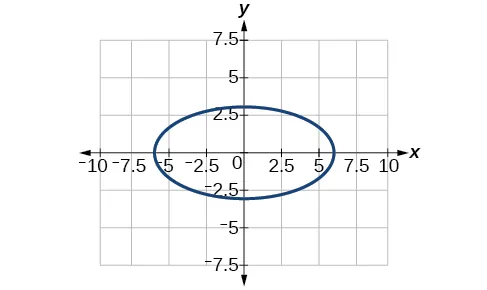7.

center: $( −2,−2 ); ( −2,−2 );$ vertices: $( 2,−2 ),( −6,−2 ),( −2,6 ),( −2,−10 ); ( 2,−2 ),( −6,−2 ),( −2,6 ),( −2,−10 );$ foci: $( −2,−2+4 3 , ),( −2,−2−4 3 ) ( −2,−2+4 3 , ),( −2,−2−4 3 )$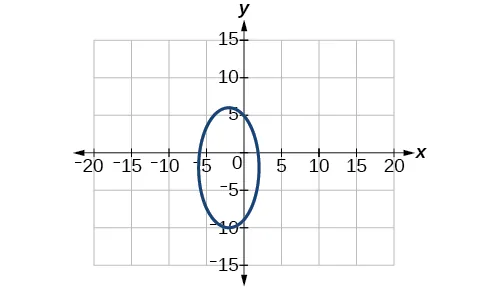9.

$x 2 25 + y 2 16 =1 x 2 25 + y 2 16 =1$

11.

Approximately 35.71 feet

13.

$( y+1 ) 2 4 2 − ( x−4 ) 2 6 2 =1; ( y+1 ) 2 4 2 − ( x−4 ) 2 6 2 =1;$ center: $( 4,−1 ); ( 4,−1 );$ vertices: $( 4,3 ),( 4,−5 ); ( 4,3 ),( 4,−5 );$ foci: $( 4,−1+2 13 ),( 4,−1−2 13 ) ( 4,−1+2 13 ),( 4,−1−2 13 )$

15.

$( x−2 ) 2 2 2 − ( y+3 ) 2 ( 2 3 ) 2 =1; ( x−2 ) 2 2 2 − ( y+3 ) 2 ( 2 3 ) 2 =1;$ center: $( 2,−3 ); ( 2,−3 );$ vertices: $( 4,−3 ),( 0,−3 ); ( 4,−3 ),( 0,−3 );$ foci: $( 6,−3 ),( −2,−3 ) ( 6,−3 ),( −2,−3 )$

17.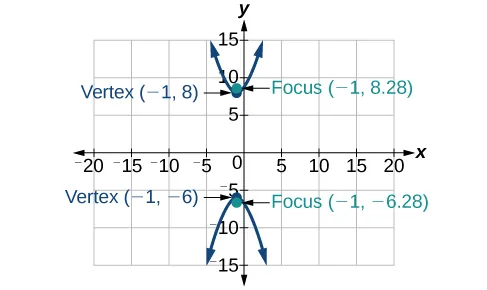19.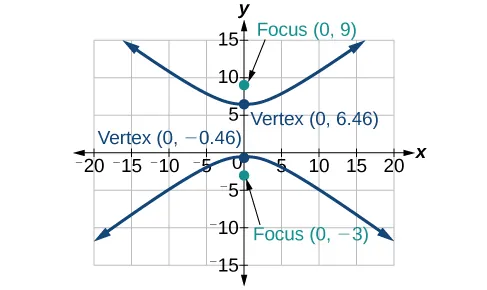21.

$( x−5 ) 2 1 − ( y−7 ) 2 3 =1 ( x−5 ) 2 1 − ( y−7 ) 2 3 =1$

23.

$( x+2 ) 2 = 1 2 ( y−1 ); ( x+2 ) 2 = 1 2 ( y−1 );$ vertex: $( −2,1 ); ( −2,1 );$ focus: $( −2, 9 8 ); ( −2, 9 8 );$ directrix: $y= 7 8 y= 7 8$

25.

$( x+5 ) 2 =( y+2 ); ( x+5 ) 2 =( y+2 );$ vertex: $( −5,−2 ); ( −5,−2 );$ focus: $( −5,− 7 4 ); ( −5,− 7 4 );$ directrix: $y=− 9 4 y=− 9 4$

27.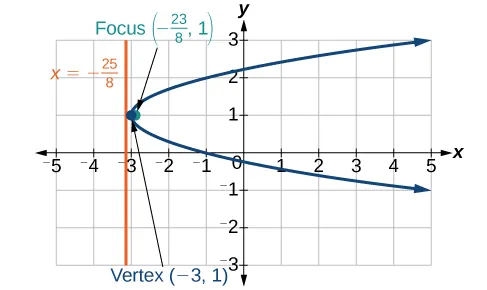29.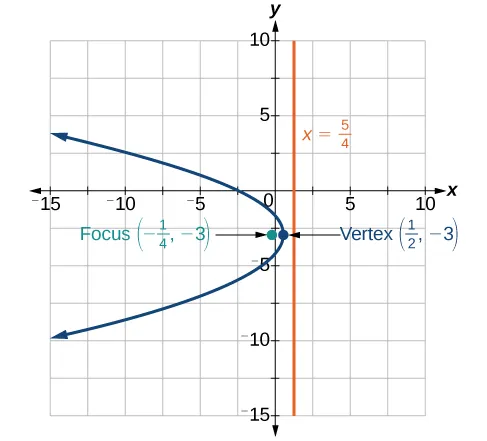31.

$( x−2 ) 2 =( 1 2 )( y−1 ) ( x−2 ) 2 =( 1 2 )( y−1 )$

33.

$B 2 −4AC=0, B 2 −4AC=0,$ parabola

35.

$B 2 −4AC=−31<0, B 2 −4AC=−31<0,$ ellipse

37.

$θ= 45 ∘ , x ′ 2 +3 y ′ 2 −12=0 θ= 45 ∘ , x ′ 2 +3 y ′ 2 −12=0$

39.

$θ= 45 ∘ θ= 45 ∘$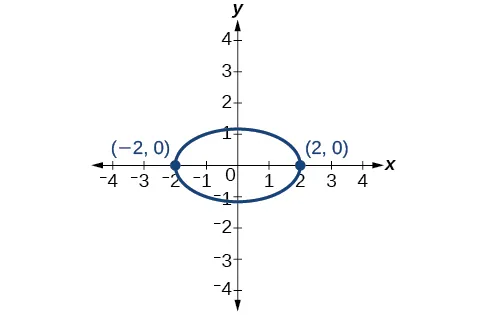41.

Hyperbola with $e=5 e=5$ and directrix $2 2$ units to the left of the pole.

43.

Ellipse with $e= 3 4 e= 3 4$ and directrix $1 3 1 3$ unit above the pole.

45.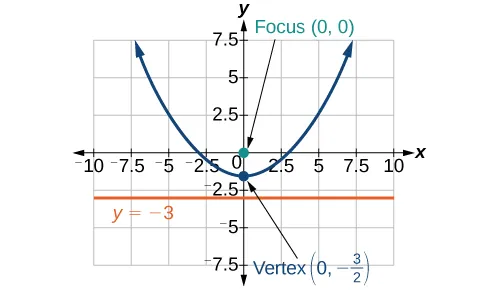47.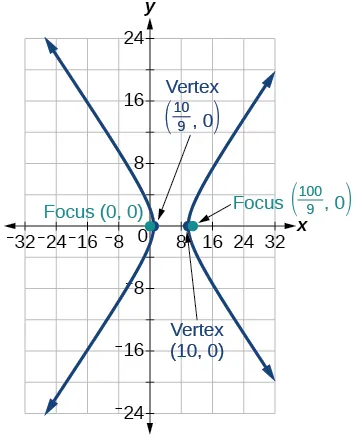49.

### Practice Test

1.

$x 2 3 2 + y 2 2 2 =1; x 2 3 2 + y 2 2 2 =1;$ center: $( 0,0 ); ( 0,0 );$ vertices: $( 3,0 ),( –3,0 ),( 0,2 ),( 0,−2 ); ( 3,0 ),( –3,0 ),( 0,2 ),( 0,−2 );$ foci: $( 5 ,0 ),( − 5 ,0 ) ( 5 ,0 ),( − 5 ,0 )$

3.

center: $( 3,2 ); ( 3,2 );$ vertices: $( 11,2 ),( −5,2 ),( 3,8 ),( 3,−4 ); ( 11,2 ),( −5,2 ),( 3,8 ),( 3,−4 );$ foci: $( 3+2 7 ,2 ),( 3−2 7 ,2 ) ( 3+2 7 ,2 ),( 3−2 7 ,2 )$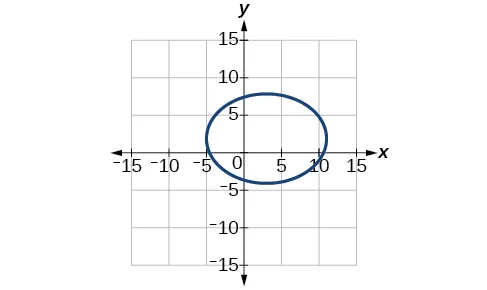5.

$( x−1 ) 2 36 + ( y−2 ) 2 27 =1 ( x−1 ) 2 36 + ( y−2 ) 2 27 =1$

7.

$x 2 7 2 − y 2 9 2 =1; x 2 7 2 − y 2 9 2 =1;$ center: $( 0,0 ); ( 0,0 );$ vertices $( 7,0 ),( −7,0 ); ( 7,0 ),( −7,0 );$ foci: $( 130 ,0 ),( − 130 ,0 ); ( 130 ,0 ),( − 130 ,0 );$ asymptotes: $y=± 9 7 x y=± 9 7 x$

9.

center: $( 3,−3 ); ( 3,−3 );$ vertices: $( 8,−3 ),( −2,−3 ); ( 8,−3 ),( −2,−3 );$ foci: $( 3+ 26 ,−3 ),( 3− 26 ,−3 ); ( 3+ 26 ,−3 ),( 3− 26 ,−3 );$ asymptotes: $y=± 1 5 (x−3)−3 y=± 1 5 (x−3)−3$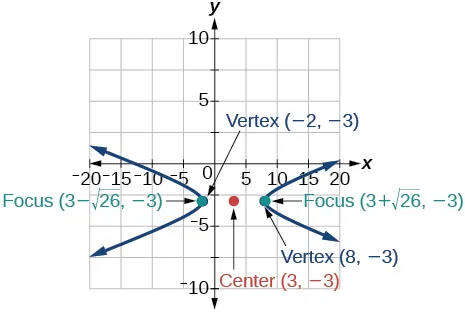11.

$( y−3 ) 2 1 − ( x−1 ) 2 8 =1 ( y−3 ) 2 1 − ( x−1 ) 2 8 =1$

13.

$( x−2 ) 2 = 1 3 ( y+1 ); ( x−2 ) 2 = 1 3 ( y+1 );$ vertex: $( 2,−1 ); ( 2,−1 );$ focus: $( 2,− 11 12 ); ( 2,− 11 12 );$ directrix: $y=− 13 12 y=− 13 12$

15.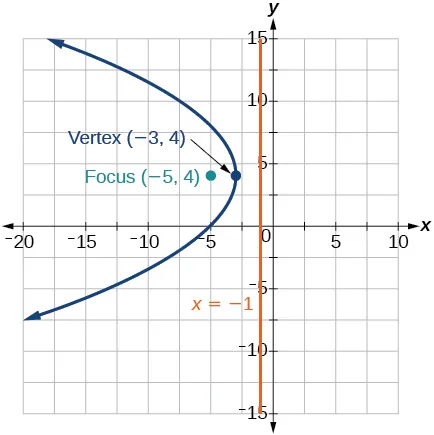17.

Approximately $8.49 8.49$ feet

19.

parabola; $θ≈ 63.4 ∘ θ≈ 63.4 ∘$

21.

$x ′ 2 −4 x ′ +3 y ′ =0 x ′ 2 −4 x ′ +3 y ′ =0$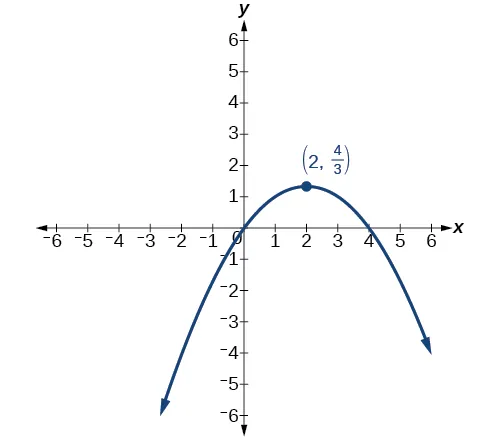23.

Hyperbola with $e= 3 2 , e= 3 2 ,$ and directrix $5 6 5 6$ units to the right of the pole.

25.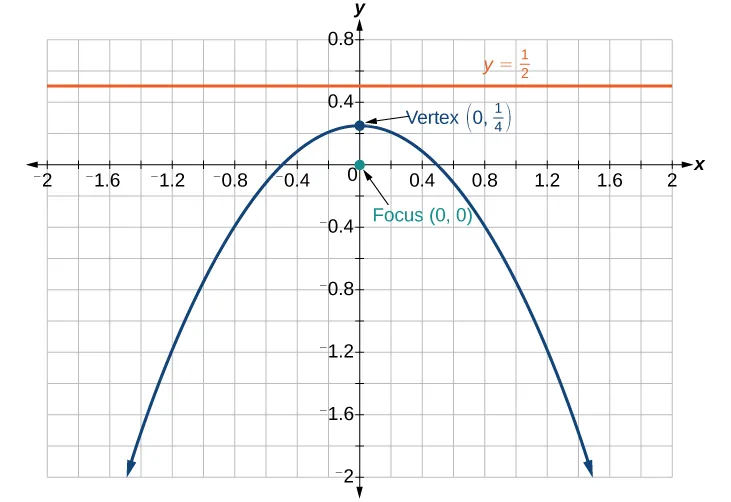Order a print copy

As an Amazon Associate we earn from qualifying purchases.